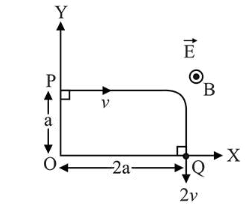# A charged particle of mass ' m ' and charge ' q 'Question:

A charged particle of mass ' $m$ ' and charge ' $q$ ' moving under the influence of uniform electric field $E \hat{i}$ and a uniform magnetic field $B \vec{k}$ follows a trajectory from point $\mathrm{P}$ to $\mathrm{Q}$ as shown in figure. The velocities at $\mathrm{P}$ and $\mathrm{Q}$ are respectively, $v \vec{i}$ and $-2 v \vec{j}$. Then which of the following statements $(A, B, C, D)$ are the correct? (Trajectory shown is schematic and not to scale)(A) $\mathrm{E}=\frac{3}{4}\left(\frac{m v^{2}}{q a}\right)$

(B) Rate of work done by the electric field at $\mathrm{P}$ is $\frac{3}{4}\left(\frac{m v^{2}}{a}\right)$

(C) Rate of work done by both the fields at $\mathrm{Q}$ is zero

(D) The difference between the magnitude of angular momentum of the particle at $\mathrm{P}$ and $\mathrm{Q}$ is 2 mav.

1. (1) $(\mathrm{A}),(\mathrm{C}),(\mathrm{D})$

2. (2) (B), (C), (D)

3. (3) (A), (B), (C)

4. (4) (A), (B), (C), (D)

Correct Option: , 3

Solution:

(3) (A) By work energy theorem

$W_{m g}+W_{\text {ele }}=\frac{1}{2} m(2 v)^{2}-\frac{1}{2} m(v)^{2}$

$0+q E_{0} 2 a=\frac{3}{2} m v^{2}$

$\Rightarrow E_{0}=\frac{3}{4} \frac{m v^{2}}{q a}$

(B) Rate of work done at $P=$ power of electric force

$=q E_{0} V=\frac{3}{4} \frac{m v^{3}}{a}$

(C) At, Q, $\frac{d w}{d t}=0$ for both the fields

(D) The difference of magnitude of angular momentum of the particle at $P$ and $Q$,

$\Delta \vec{L}=(-m 2 v 2 a \hat{k})-(-m v a \hat{k})$

$|\Delta \vec{L}|=3 \mathrm{mva}$